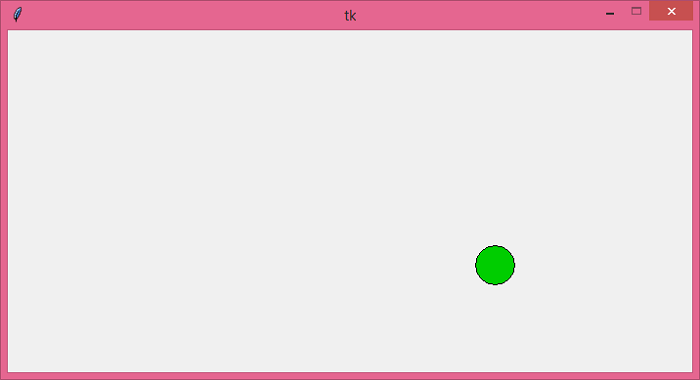# Moving balls in Tkinter Canvas

Tkinter is a standard Python library which is used to create GUI-based applications. To create a simple moving ball application, we can use the Canvas widget which allows the user to add images, draw shapes, and animating objects. The application has the following components,

• A Canvas widget to draw the oval or ball in the window.

• To move the ball, we have to define a function move_ball(). In the function, you have to define the position of the ball that will constantly get updated when the ball hits the canvas wall (left, right, top, and bottom).

• To update the ball position, we have to use canvas.after(duration, function()) which reflects the ball to change its position after a certain time duration.

• Finally, execute the code to run the application.

## Example

# Import the required libraries
from tkinter import *

# Create an instance of tkinter frame or window
win=Tk()

# Set the size of the window
win.geometry("700x350")

# Make the window size fixed
win.resizable(False,False)

# Create a canvas widget
canvas=Canvas(win, width=700, height=350)
canvas.pack()

# Create an oval or ball in the canvas widget
ball=canvas.create_oval(10,10,50,50, fill="green3")

# Move the ball
xspeed=yspeed=3

def move_ball():
global xspeed, yspeed

canvas.move(ball, xspeed, yspeed)
(leftpos, toppos, rightpos, bottompos)=canvas.coords(ball)
if leftpos <=0 or rightpos>=700:
xspeed=-xspeed

if toppos <=0 or bottompos >=350:
yspeed=-yspeed

canvas.after(30,move_ball)

canvas.after(30, move_ball)

win.mainloop()

## Output

Running the above code will display an application window that will have a movable ball in the canvas.